# Concrete pedestal

The carpenters made wooden mold on a concrete pedestal in the shape of a cube with edge 2 meter long. What is the area in which the concrete touches wooden molds? (No lid or bottom)

Result

S =  16 m2

#### Solution:Leave us a comment of example and its solution (i.e. if it is still somewhat unclear...):

Showing 0 comments:Be the first to comment!## Next similar examples:

1. Carpenter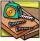You want to cut long board into smaller pieces. Carpenter said that one cut of the board into two pieces will cost you 100 cents. But you need to cut the board into 9 parts. How much you pay?
2. CubeHow many times increases the surface area of a cube with edge 23.4 cm if the length of the edge doubled?
3. Cube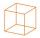Calculate the surface cube with edge 11 dm.
4. The cube - simpleCalculate the surface of the cube measuring 15 centimeters.
5. Cube surface areaWall of cube has content area 99 cm square. What is the surface of the cube?
6. Cube 2How many times will increase if the surface area of the cube if we triple length of its edge?
7. Addition of Roman numbersAdded together and write as decimal number: LXVII + MLXIV
8. MultiplesFind all multiples of 10 that are larger than 136 and smaller than 214.
9. Computer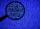A line of print on a computer contains 64 characters (letters, spacers or other chars). Find how many characters there are in 7 lines.
10. Number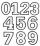Which number is 17 times larger than the number 6?
11. ProductResult of the product of the numbers 1, 2, 3, 1, 2, 0 is:
12. The tap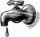For one day flows 148 l of water out of the tap. How much water will flow out for 3/4 day?
13. Jan and DanJan and Dan had the same money. Jan bought 5 workbooks and left him 15 CZK. Dan 6 and left him nothing. How much money have in total?
14. Seeds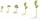Of the 72 wet seeds 7/8 germinated. How many seeds germinate?
15. Unknown number 2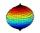I think the number. When he reduces it four times, I'll get 11. What number am I thinking?
16. Written numberPlace+values x ten thousands =30 thousands
17. How manzBy how many is the product of the numbers 328 and 7 greater than its sum?